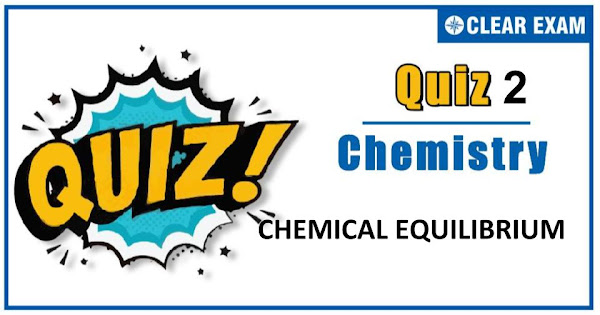## [LATEST]\$type=sticky\$show=home\$rm=0\$va=0\$count=4\$va=0

The one subject in NEET which is candidates who can easily attain good marks is Chemistry. That's the reason, often, one doesn’t pay notice and choose to compromise it. But if one wants to rank above others, the tip is to be thorough with NEET chemistry concepts. The understanding of reactions and definite basic understanding is what requires major attention in Chemistry but once done it only gets simpler from there. The main focus on the to-do list should be on getting a hang of the NCERT syllabus of NEET chemistry.
.

Q1. For the reversible reaction, N2(g)+3H2(g)⇌2NH3(g) at 500℃, the value of Kp is 1.44×10(-5). When partial pressure is measured in atmospheres.
The corresponding value of Kc with concentration in mol L(-1) is
•  1.44×10-5⁄(0.082×773)-3
•  1.44×10-5⁄(0.082×500)-2
•  1.44×10-5⁄(0.314×773)2
•  1.44×10-5⁄(0.082×773)-2
Solution
(d) For the reversible reaction, N2 (g)+3H2(g)⇌2NH3(g) ∆ng=2-(1+3) =2-4=-2 R=0.0821 L atm K(-1) mol(-1) T=500+273=773 K Kp=Kc(RT)(∆ng) Kp=Kc×(RT)(-2) or Kc=Kp/(RT)(-2) =(1.44×10(-5))/(0.082×773)(-2) or (1.44×10(-5))⁄(0.082×773)(-2)

Q2.Le-Chatelier principle is applicable only to a
•  System in equilibrium
•  System not in equilibrium
•  Homogeneous reaction
•  Heterogeneous reaction
Solution
(a)
Q3.  The expression for the solubility product of Ag2CO3 will be
•  Ksp s2
•  Ksp 4s3
•  Ksp 27s4
•  Ksp s
Solution
(b) Ag2CO3(s)⇌2Ag++CO3^(2-) s 2s s Ksp=[Ag+ ]2 [CO3(2-)]=(2s)2.s ∴ Ksp=4s3

Q4. The combination of H+ and OH- to produce H2O is called:
•  Hydrolysis
•  Neutralization
•  Dehydration
•  Dehydrohalogenation
Solution
(b) H++OH-⟶H2O;∆H=13.7 kcal is neutralization process.

Q5.The pKa of HCN is 9.30. The pH of a solution prepared by mixing 2.5 moles of KCN and 2.5 moles of KCN and 2.5 moles of HCN in water and making up the total volume of 500 mL, is
•  9.30
•  7.30
•  10.30
•  8.30
Solution
a) pH=pKa+log⁡([KCN]/[HCN]) =9.3+log⁡(2.5/2.5)=9.30

Q6.If in the reaction N2O4⇌2NO2,α is degree of dissociation of N2O4, then the number of molecules at equilibrium will be:
•  3
•  1
•  (1-α)2
•  (1+α)
Solution
d) N2O4⇌2NO2 1 0 (1-α) 2α ∴ Total mole at equilibrium =1-α+2α=1+ α

Q7.A gaseous phase reaction is allowed to attain equilibrium as A(g)⇌B(g)+C (g) at constant pressure P. The partial pressure of A at equilibrium is P/2. The value of equilibrium constant KP is∶
•  P/2
•  P/4
•  P/6
•  P/8
Solution
d) A ⇌ B+C PA'=(a-x)P/((a+x))=P/2 ∴ x=a/3 ∴ Kp=(x.x)/(a-x) x [p/(a+x)]1 =(a/3 ×a /3 × P)/(a2-a2/9) = P/8

Q8.5 moles of SO2 and 5 moles of O2 are allowed to react. At equilibrium, it was found that 60% of SO2 is used up. If the partial
pressure of the equilibrium mixture is one atmosphere, the partial pressure of O2 is
•  0.82 atm
•  0.52 atm
•  0.21 atm
•  0.41 atm
Solution
d) 2SO2 (g) + O2(g) ⇌ 2SO3 (g) Initial 5 5 0 At equilibrium (5-3) (5-1.5) 3 =2 =3.5 ∴pO2=(3.5×1)/8.5=0.41atm

Q9.The value of Kp for the following reaction 2H2S(g)⇌2H2(g)+S2(g),is 1.2×10(-2) at 106.5℃. The value of Kc for this reaction is
•  =1.2×10^(-2)
•   <1.2×10^(-2)
•  >1.2×10^(-2)
•  None of these
Solution
(b) Kp=Kc(RT)(∆ng ) Here, ∆ng=1 Thus, Kc will be less than Kp

Q10. If NaOH is added to a solution of acetic acid:
•  H+ ions increases
•   pH decreases
•   [C2H3O2]- increases
•   [HC2H3O2] increases
Solution
c) Dissociation of acetic acid increases as, CH3COOH+NaOH⟶CH3COONa+H2O Concentration of mixture#### Written by: AUTHORNAME

AUTHORDESCRIPTION## Want to know more

Please fill in the details below:

## Latest NEET Articles\$type=three\$c=3\$author=hide\$comment=hide\$rm=hide\$date=hide\$snippet=hide

Name

ltr
item
BEST NEET COACHING CENTER | BEST IIT JEE COACHING INSTITUTE | BEST NEET & IIT JEE COACHING: CHEMICAL EQUILIBRIUM QUIZ-2
CHEMICAL EQUILIBRIUM QUIZ-2
https://1.bp.blogspot.com/--3DQrXlFeP8/X5mdan1rDnI/AAAAAAAAGW0/Tn1hC6Htx2wRAP-ABSG4pjbr4sdHfJ9iQCLcBGAsYHQ/s600/Quiz%2BImage%2B20.jpg
https://1.bp.blogspot.com/--3DQrXlFeP8/X5mdan1rDnI/AAAAAAAAGW0/Tn1hC6Htx2wRAP-ABSG4pjbr4sdHfJ9iQCLcBGAsYHQ/s72-c/Quiz%2BImage%2B20.jpg
BEST NEET COACHING CENTER | BEST IIT JEE COACHING INSTITUTE | BEST NEET & IIT JEE COACHING
https://www.cleariitmedical.com/2020/10/chemical-equilibrium-quiz-2.html
https://www.cleariitmedical.com/
https://www.cleariitmedical.com/
https://www.cleariitmedical.com/2020/10/chemical-equilibrium-quiz-2.html
true
7783647550433378923
UTF-8

STAY CONNECTED